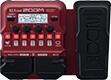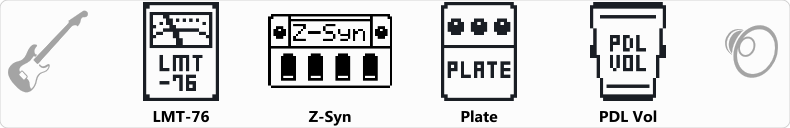# Disco

Discussion in 'Zoom B1/B1X Four' started by Jailton Sampaio, Sep 4, 2020.

1. ### Jailton SampaioNew Member

DiscoDevice: Zoom B1X Four
Firmware: 1.10

Name on device: Disco
Optimized for: Phones/Speaker

Effects chain:Play Disco 70´s

Effect: "LMT-76" (Dynamics), active - "yes"
"Input" = 40
"Ratio" = 4:1
"REL" = 20
"Output" = 38

Effect: "Z-Syn" (Sfx), active - "yes"
"FREQ" = 2
"Range" = 6
"Decay" = 35
"RESO" = 18
"Wave" = 0
"Tone" = 7
"BAL" = 41
"VOL" = 80
"Frequency" = 0
"Range" = 0
"Decay" = 0
"Resonance" = 0
"Wave" = SAW
"Tone" = 0
"Balance" = 0
"Volume" = 0

Effect: "Plate" (Reverb), active - "yes"
"PreD" = 9
"Decay" = 40
"Mix" = 14
"Tail" = Off

Effect: "PDL Vol" (Pedal), active - "yes"
"Volume" = 100
"Min" = 0
"Max" = 100
"Curve" = A

Patch Volume: 100